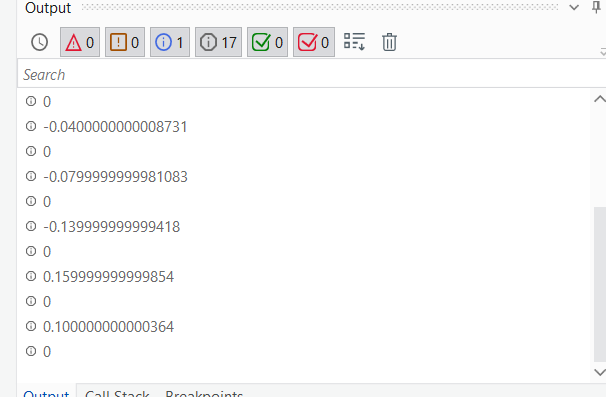# Linq If Condition

Hi Team,

Attaching an excel, i want that if column R is lessthan 1 or greater than -1 then replace it with 0.
OutputFile.xlsx (1.3 MB)

@Quenton_Wayne_Rebello Any Help?

i want this thing with the use of LINQ.

we suggest to invest some time into LINQ learning:

[HowTo] LINQ (VB.Net) Learning Catalogue - Help / Something Else - UiPath Community Forum

Understanding the 6 Debugging Panels of UiPath in the easiest way possible! - News / Tutorials - UiPath Community Forum

For coversions have a look at:
CDbl(…
Convert.ToDouble(…
Double.Parse(…

Please give some more clarification or show the sample required output…

i have a column which has values as -0.05,5.0,0.3, 6,10.3 etc etc.,
i want that if any value which is less than 1 and greater than -1 i.e. lies between -1 and 1, i want to update it with 0

@Quenton_Wayne_Rebello any help?

Assign
CurrentRow(“Difference between Before Tax Amount”)=If((Math.Abs(CDbl(CurrentRow(“Difference between Before Tax Amount”).ToString)))<1, “0”, CurrentRow(“Difference between Before Tax Amount”).ToString)i will again have to use it in for each loop, i want it to use it through linq, because my data is huge.

write the new dt based on condition using below linq

syntax : DT.AsEnumerable.Where(Function(r) if(double.parse(r(column name).ToString) < 1 or double.parse(r(columnname).ToString) > -1 ,True,False)).CopyToDataTable

and write the new dt values as zero using below linq

syntax : New DT.AsEnumerable.select(Function(r) New DT.Clone.LoadDataRow(New Object(){r(0).ToString,“0”},false)).copytodatatable

use invoke code activity
where in_dt=inputdatatable

For Each r As DataRow In in_dt.AsEnumerable
r(“Difference between Before Tax Amount”)=If((Math.Abs(CDbl(r(“Difference between Before Tax Amount”).ToString)))<1, “0”, r(“Difference between Before Tax Amount”).ToString)
Next r

Hey @yash.choursia,

You can try the following code

``````(From row In dt.AsEnumerable
Let updateDiffer = If(CDbl(row(17).ToString) > -1.00, If(CDbl(row(17).ToString) < 1.00,"0",row(17).ToString),row(17).ToString)
Select ra = row.ItemArray.Take(17).Append(updateDiffer).Concat(row.ItemArray.Skip(18)).ToArray